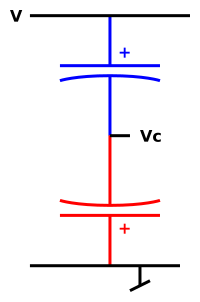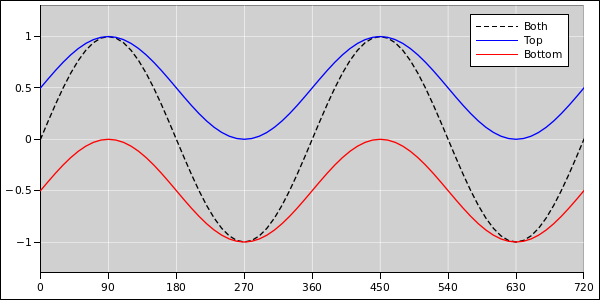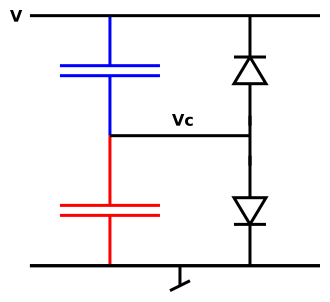[Contents]

Non-Polarized Capacitor
John Denker

Question: Will two electrolytics back-to-back work as a non-polarized capacitor?

Answer: Yes. This is standard practice. Very common.Figure 1: Non-Polarized Capacitor

This technique was mentioned in class when I was a freshman. They spent about ten seconds on it. They said “it works” and left us to figure out the details, if-and-when we needed to. The remaining questions include:

• How does this work?
• In particular, what happens to the weird floating node?

## 1  Discussion

1.
Since the system is linear, we can do the DC analysis and AC analysis separately. This is a basically a Fourier series with only two terms, DC and AC.

2.
As for the AC component: Obviously the pair works as a capacitive voltage divider. So if the AC voltage across the pair is V, each capacitor separately sees (VVc) = (Vc−0) = V/2 @AC ... where these are the instantaneous voltages, and we are talking about the AC component only.

3.
As for the DC component: It is helpful to compare the capacitive voltage divider to a resistive voltage divider, as diagrammed in figure 2.Figure 2: Capacitive versus Resistive Divider

At DC we need to worry about the floating node. (At AC it doesn’t float, because the capacitors have nonzero AC conductance.)

During normal operation, the floating node has a voltage Vc that is offset from the corresponding Vr by an amount −⌈V⌉/2, where ⌈V⌉ is the peak of the waveform. That means the total (AC plus DC) voltage drops are:

 top: (V − Vc) in the range from −⌈V⌉/2 + ⌈V⌉/2 to ⌈V⌉/2 + ⌈V⌉/2 (1a) bottom: (Vc − 0) in the range from −⌈V⌉/2 − ⌈V⌉/2 to ⌈V⌉/2 − ⌈V⌉/2 (1b) both: (V − 0) in the range from −⌈V⌉ to ⌈V⌉ (1c)

Equation 1c is obtained by summing the two previous equations. The waveforms are shown in figure 3.Figure 3: The Waveforms

.

4.
This works because if you reverse-bias an electrolytic capacitor, it does not immediately explode; it just leaks current across the capacitor gap. You don’t want to make a habit of this, but you’re allowed to do it once, to drive down the voltage on the floating node.
Note: I use the word gorge in what follows, for reasons discussed in reference 1. The more-common but more-confusing term would be “charge”.

In more detail: Suppose we start out with Vc=0, which is not where it should be. It won’t stay there. During the transient, if the input voltage V is high, the bottom capacitor leaks, putting gorge on the top capacitor (but not the bottom), lowering Vc. If V is low, the top capacitor leaks, putting gorge on the bottom capacitor (but not the top), again lowering Vc.

Both capacitors operate with the correct polarity at all times after the initial transient.

5.
We are assuming the two capacitors have the same capacitance and the same voltage rating.

The capacitance of the pair is half the capacitance of each component, in accordance with Ohm’s law in the usual way.

The voltage rating of the pair is equal to the voltage rating of each component. At the positive peak the top capacitor has to withstand the entire input voltage; 180 degrees later the bottom capacitor has to, as you can see in figure 3.

6.
I love a good symmetry argument as much as the next guy, but it is worth noting that the right answer here does not have the symmetry you might naïvely expect. The resistive divider is symmetric, but the capacitive divider is not. The midpoint of the resistive divider is V/2 (AC and DC) but the midpoint of the capacitive divider is not.

7.
Beware: Floating nodes are weird. Sometimes it’s OK to build something with a floating node, but you have to analyze it carefully.

The following may help; if not, just ignore it: Suppose we temporarily freeze the input voltage at V=0. Then the floating node has some capacitance to ground. Consider the two (−) plates of the capacitors as forming one side of a double-sized capacitor, while the two (+) plates form the other side. That is to say, the floating node sees two capacitors in parallel.

More generally, suppose we unfreeze V, and instead assume V is driven by a low-impedance source. Then the floating node sees two capacitors in parallel, with the other terminal tied to V/2.

Leakage puts some gorge on this double-sized capacitor. In this sense we can speak of gorge “on” the floating node.

8.
The leakage current flows through the capacitor, so when it is adding positive charge to one plate it is removing positive charge from the other plate, so the overall effect is gorge, not free charge, not unbalanced charge. Kirchhoff’s so-called laws2 are not violated.

Keep in mind that one capacitor is leaking while the other is not, so the current through the pair cannot be divided into “leakage” current and “non-leakage” current.

It may help to imagine each real capacitor as an ideal capacitor plus a separate leakage model, as shown in figure 4. This helps make the point that the leakage phenomenon is not magic; it just gorges and disgorges the capacitors in the usual prosaic way.Figure 4: Ideal Capacitors + Leakage Model

9.
Beware: I tried googling for this and found a lot of spectacularly bad explanations.

## 2  References

1.
John Denker, “Gorge versus Charge”
www.av8n.com/physics/gorge-vs-charge.htm
2.
John Denker, “Kirchhoff’s Circuit Laws”
www.av8n.com/physics/kirchhoff-circuit-laws.htm
3.
John Denker, “Capacitance”
www.av8n.com/physics/capacitance.htm
[Contents]